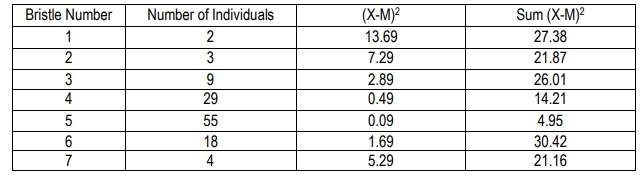## Genetics

Learn the toughest concepts covered in biology with step-by-step video tutorials and practice problems by world-class tutors

20. Quantitative Genetics

# Mathematical Measurements

1
concept

## Mathematical Measurements11m
Play a video:
2
Problem

The table shows a distribution of bristle numbers in a Drosophila population. What is the variance?3
Problem

Using the variance calculated in problem #2, what is the standard deviation?